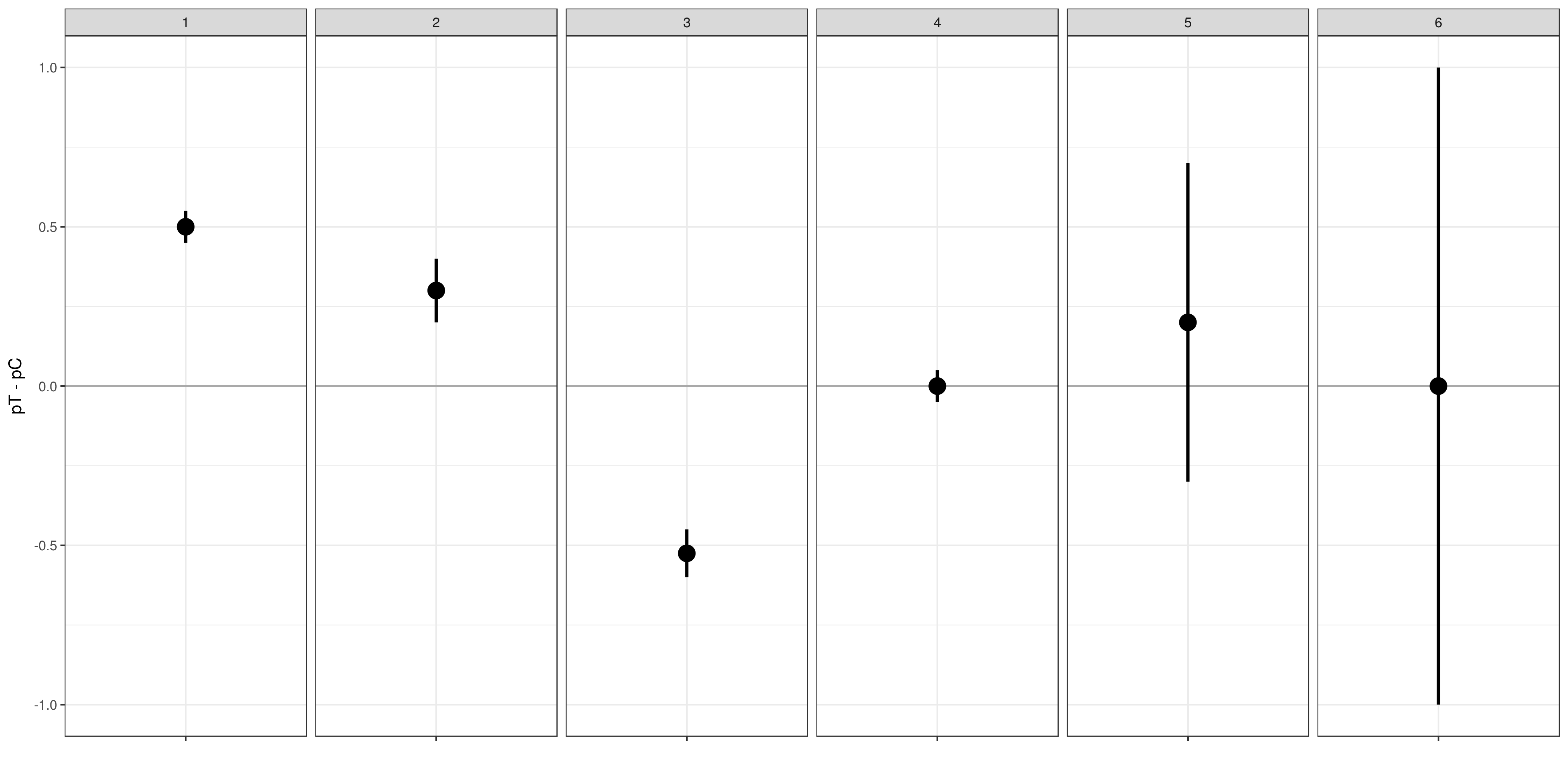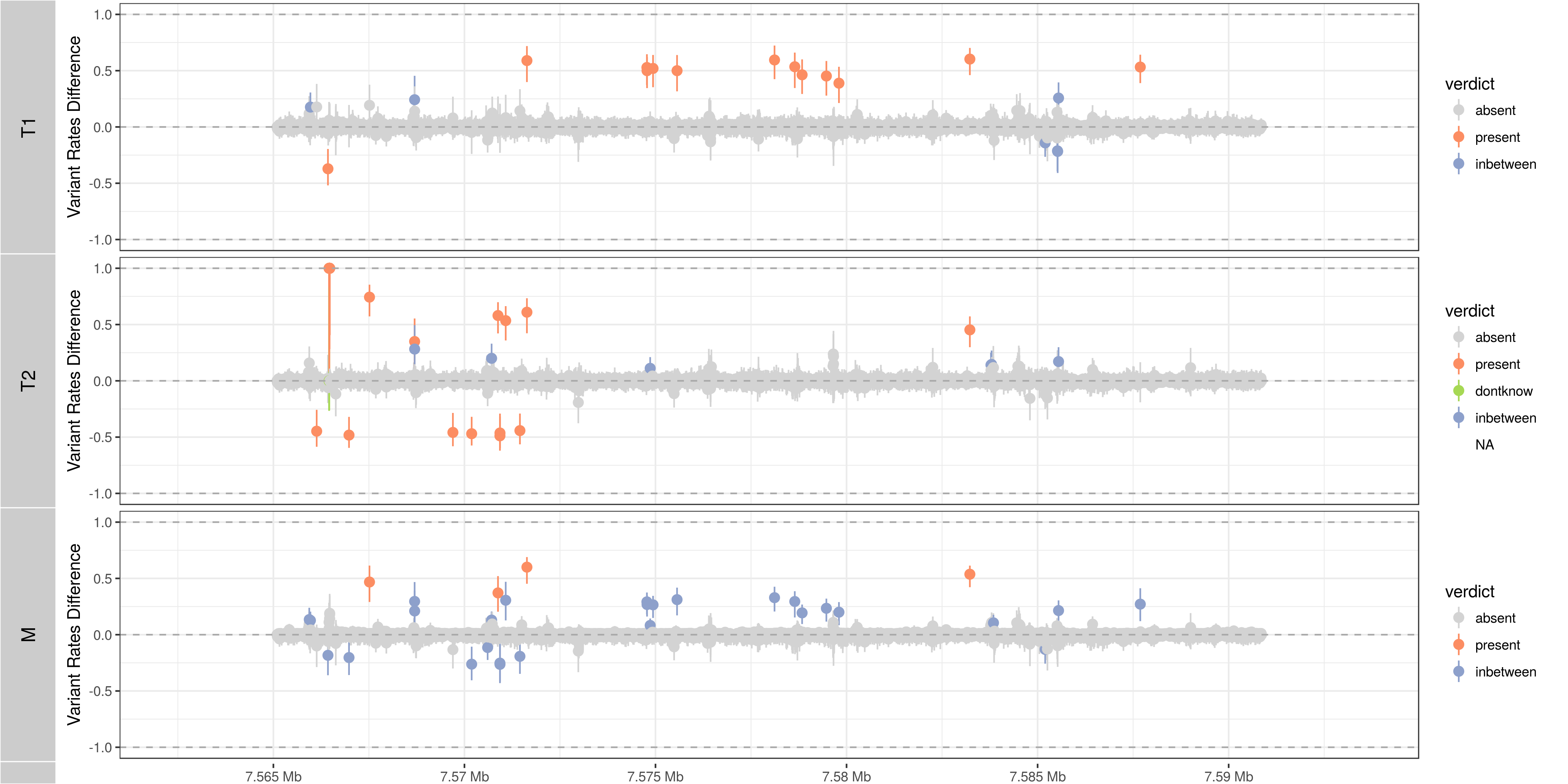# Comparative Identification and Assessment of Single Nucleotide Variants Through Shifts in Base Call Frequencies

Julian Gehring, Simon Anders, Bernd Klaus (EMBL Heidelberg)

## 1 Motivation

The advances in high-throughput nucleotide sequencing have lead to new possibilities of identifying genomic alterations, which is of particular interest for cancer research. While the detection of genomic variants is often conducted by comparing against a population-based reference sequence (such as the human GRCh 37), in the investigation of tumors a comparison between matched samples is predominantly pursued. Considering a test sample $$T$$ and a control sample $$C$$, the aim is to identify single nucloetide variants (SNVs) which show significant changes between the two. Typically, this is applied for comparing a tumor sample against a matched normal/constitutive sample of the same patient, focusing on alterations that may have occurred in the transition to the cancerous tissue, and therefore are often termed somatic variants. However, a broader range of questions can be addressed by extending the focus to include genomic changes of temporally or spatially separated samples to each other, to investigate tumor evolution and subclonal shifts .

Extending the scope of comparative variant analyses in a cancer related setting may require an accompanying change in definitions. In the classical tumor versus normal setting, a somatic event is characterized by both the presence of the variant in the tumor and the absence in the matched normal . This definition is not satisfactory for comparing different tumor stages against each other. If we imagine two stages of a tumor haboring multiple subclones, variants may be present in both stages with changing abundances. Here, the classical definition of a somatic variant would result in missing the event. In the following, we will therefore focus on estimating the shift in variant allele frequencies between a test and a control sample. The classical somatic definition requiring the variant to be absent in the control constitutes a special case of this.

Numerous approaches focus on more narrowly defined somatic comparisons, motivated by scientific questions in the field of cancer genomics  . Most existing methods do not allow an explicit statement about the evidence for the absence of a putative variant in a sample, and a clear distinction is needed whether a negative call results from a true negative site or the lack of statistical power to detect it. As an example, imagine a variant calling approach using a hypothesis test with the null hypothesis that the variant frequencies at a locus are identical for both samples. While a significant p-value provides evidence for the presence of a variant, inverting this argument does not work: A non-significant p-value can both be indicative of the absence or insufficient power. Furthermore, most methods return only a point estimate for the variant frequency in the tumor, without stating the confidence of the estimate. However, this information would be critical to reliably differentiate between variants arising from competing subclonal populations.

With the Rariant package, we pursue a generalized approach for identifying and quantifying SNV alterations from high-throughput sequencing data in comparative settings. By focusing on shifts of the non-consensus base call frequencies, events like loss of heterozygosity and clonal expansions in addition to somatic variants can be detected. In the following, we will 1) outline the methodological framework, 2) provide an example workflow on how to obtain a call set starting from short-read alignments, and 3) illustrate potential benefits of the methods on a biological cancer data set. This methodology can be used for a high performance identification of variant sites as well as to quantitatively assess the presence or absence in comparisons between matched samples.

## 2 Methodology

### 2.1 Comparative shift of non-consensus base call frequencies

Starting with a set of aligned reads we observe the sequencing depth $$N^{S}_{i}$$ and the number of non-consensus base calls $$K^{S}_{i}$$ for a sample $$S$$ at position $$i$$. Non-consensus is here defined as base calls that differ from the consensus sequence, which can be either the reference sequence or, in a comparative setting, the most abundant base call in the control sample. The non-consensus rate $$p^{S}_{i}$$, which we assume to be binomially distributed, can then be estimated as

$$\hat{p}^{S}_{i} = \frac{K^{S}_{i}}{N^{S}_{i}}$$

for sites with $$N^{S}_{i} > 0$$. Since $$K^{S}_{i} \leq N^{S}_{i}$$, the rates are bound to the range $$[0,1]$$.

The true non-consensus rate

$$p^{S}_{i} = v^{S}_{i} + e^{S}_{i}$$

comprises the presence of a putative variant with a frequency $$v^{S}_{i}$$ and a technical error rate $$e^{S}_{i}$$. In order to detect and describe the change in the variant frequency, we focus on the shift $$d_{i}$$ in non-consensus rates as the difference of the rates between the test and control samples, which we estimate as

$$\hat{d}_{i} = \hat{p}^{T}_{i} - \hat{p}^{C}_{i}.$$

If we assume that the true site-specific technical error rates are identical between the two matched samples , the difference of the rates yields an unbiased estimate for the change in the variant frequency. Thus, positions not haboring biological alterations will result in $$\hat{d}_{i} \approx 0$$.

### 2.2 Confidence intervals

Distinguishing biological variants from noise requires knowledge about the variance of the point estimate $$\hat{d_{i}}$$. By constructing a confidence interval (CI) for $$d_{i}$$ with confidence level $$\beta$$ , we assess the certainty of the estimated shift in non-consensus frequencies. The probability of the true value being outside the confidence interval is less than $$\alpha = 1 - \beta$$. This is in concordance with the type I or $$\alpha$$ error definition in statistical testing.

Under the assumption that the non-consensus counts $$K^{S}_{i}$$ in our samples follow binomial distributions with parameters $$p^{S}_{i}$$ and $$N^{S}_{i}$$, several methods have been established for estimating confidence intervals for the difference of two rate parameters  . The performance of an approach is generally described in terms of its coverage probability indicating the probability of a confidence interval to cover the true value (see 4.2). Coverage probabilities greater and less than the confidence level $$\beta$$ describe conservative and liberal behaviors, respectively. Due to the conservative coverage probabilities and high computational effort of exact confidence interval estimates, approximate methods are generally preferred  .

The Agresti-Caffo (AC) confidence interval 

$$\tilde{p}^{T} - \tilde{p}^{C} \pm z \sqrt{ \frac{\tilde{p}^{T} (1 - \tilde{p}^{T})} {\tilde{N}^{T}} + \frac{\tilde{p}^{C}(1 - \tilde{p}^{C})} {\tilde{N}^{C}} }$$

with

$$\tilde{p}^{X} = \frac{K^{X}+\zeta}{N^{X}+2\zeta},$$

$$\tilde{N}^{X} = N^{X} + 2\zeta,$$

$$\zeta = \frac{1}{4} z^2,$$

and $$z = z_{(1-\beta)/2}$$ as the upper $$(1-\beta)/2$$ percentile of the standard normal distribution), is an approximation of the score test-based confidence interval. Several publications emphasize the usefulness and advantages of the AC method over related approaches   .

#### 2.2.1 Decision making with confidence intervals

While the estimate for the shift in the non-consenus frequency $$\hat{d}$$ indicates the change in abundance and direction of a variant, the corresponding confidence interval gives us information about the precision and power of the estimate. Generally, wide confidence intervals will be present at sites with little statistical power, as due to low sequencing depths.

For the case that we compare a tumor to a matched normal sample, we show a set of hypothetical cases that can be distinguished by regarding the point estimate and its confidence interval:

1. Presence of a somatic, heterozygous variant
2. Presence of a somatic, subclonal variant
3. Presence of loss of heterozygosity
4. Absence of a somatic variant
5. Presence or absence of a variant cannot be distinguished due to the low certainty of the estimate
6. No power due to insufficient sequencing depthIllustrative cases of confidence intervals for somatic variant frequency estimates

### 2.3 Distinguishing event classes

Focusing on the comparative shift of non-consensus frequencies allows us to detect and distinguish different types of events. Since Rariant does not make explicit assumptions about the abundance of a potential variant in the control sample, we are further able to find clonal shifts, for example between different tumor samples, or losses of heterozygocity. Generally, gains and losses of variant alleles are characterized by positive and negative values of $$d$$, respectively. For a differentiated interpretation of the results, we classify a variant into one of four classes:

somatic
A somatic variant that does not occur in the control sample
hetero/LOH
A shift away from heterozygous SNP in the control sample
undecided
Both of the 'somatic' or 'hetero' are possible
powerless
A distinction between the two classes cannot be made due to a lack of power

The classification is based on two binomial tests for each position:

1. Somatic variants where the variant allele is not present in the control sample, rejecting a binomial test with the alternative hypothesis $$H_{1}: p^{C} > 0$$.
2. Sites with a loss of heterozygosity with a shift away from a heterozygous variant in the control sample, rejecting a binomial test with the alternative hypothesis $$H_{1}: p^{C} \neq \frac{1}{2}$$.

### 2.4 Identifying variant sites in large datasets

The method that we have described before is suited for detecting variant positions efficiently in large sequencing datasets, including whole-exome and whole-genome sequencing. For this purpose, we test for a shift in non-consensus frequencies between two samples at each genomic position individually:

1. Form the base counts table for four bases A, C, G, T from the aligned reads. In order to reduce the number of false counts, we can optionally exclude reads with low base calling quality and clip the head of each read.
2. Determine the consensus sequence: In a comparative setting, we will use the most abundant base call.
3. Calculate the sequencing depth $$N^{S}_{i}$$, mismatch counts $$K^{S}_{i}$$, and derived statistics for both samples, based on the consensus sequence (see 2.1).
4. Find potential variant sites with a Fisher's Exact Test, comparing the number of mismatching and total bases between the samples: $${K^{T}_{i}, N^{T}_{i}, K^{C}_{i}, N^{C}_{i}}$$. The p-values are corrected for multiple testing according to the Benjamini-Hochberg procedure. Only positions rejecting the null hypothesis at a significance level $$\alpha$$ are further on considered as potential variants.
5. Calculate Agresti-Caffo confidence intervals with confidence level $$\beta$$, in order to evaluate presence or absence of the variant (see 2.2).
6. Classify variant sites into the groups: somatic, LOH, undecided, and powerless (see 2.3).

## 3 Workflow

### 3.1 Multiple Sample Simulation Study

We want to further demostrate the usage and abilities of the Rariant package on a real-life data set. Due to legal and privacy issues, most human cancer sequencing data is not publicly accessible and therefore cannot serve as an example data set here. Alternatively, we conduct an analysis to mimic the characteristics of current cancer sequencing studies.

For the purpose of the analysis, we compare three samples from the 1000 Genomes project , serving as a control/normal (control) and two related test/tumor samples (test and test2). Further, we simulate a clonal mixture (mix) of the two test samples by combining their reads.

library(Rariant)

library(GenomicRanges)
library(ggbio)

tp53_region = GRanges("chr17", IRanges(7565097, 7590856))

control_bam = system.file("extdata", "platinum", "control.bam", package = "Rariant", mustWork = TRUE)
test1_bam = system.file("extdata", "platinum", "test.bam", package = "Rariant", mustWork = TRUE)
test2_bam = system.file("extdata", "platinum", "test2.bam", package = "Rariant", mustWork = TRUE)
mix_bam = system.file("extdata", "platinum", "mix.bam", package = "Rariant", mustWork = TRUE)

v_test1 = rariant(test1_bam, control_bam, tp53_region, select = FALSE)

   Warning: rbind_all() is deprecated. Please use bind_rows()

v_test2 = rariant(test2_bam, control_bam, tp53_region, select = FALSE)

   Warning: rbind_all() is deprecated. Please use bind_rows()

v_mix = rariant(mix_bam, control_bam, tp53_region, select = FALSE)

   Warning: rbind_all() is deprecated. Please use bind_rows()

v_all = GenomicRangesList(T1 = v_test1, T2 = v_test2, M = v_mix)

v_all = endoapply(v_all, updateCalls)


To better understand the evidence for the presence or absence of particular variants across samples, we plot the confidence intervals, colored according to the predicted event type, and abundance shifts for all sites of interest, colored according to the sign of the shift.

t_ci = tracks(lapply(v_all, plotConfidenceIntervals, color = "verdict")) + verdictColorScale()

print(t_ci)

   Warning: Removed 6 rows containing missing values (geom_pointrange).Confidence intervals for simulation study

t_ci = tracks(lapply(v_all, plotConfidenceIntervals, color = "eventType"))

print(t_ci)

   Warning: Removed 6 rows containing missing values (geom_pointrange).